# Disinfecting solution

How much distilled water is necessary to pour into 500 ml of 33% hydrogen peroxide solution to obtain 3% disinfecting solution?

x =  5000 ml

### Step-by-step explanation:

33/100 • 500 = 3/100 (x+500)

33/100 • 500 = 3/100•(x+500)

3x = 15000

x = 5000

Our simple equation calculator calculates it.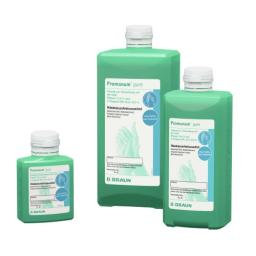Did you find an error or inaccuracy? Feel free to write us. Thank you!Tips to related online calculators
Need help to calculate sum, simplify or multiply fractions? Try our fraction calculator.
Do you have a linear equation or system of equations and looking for its solution? Or do you have a quadratic equation?
Tip: Our volume units converter will help you with the conversion of volume units.

## Related math problems and questions:

• Clogging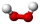How much-distilled water must the pharmacist add to 30g of a 30% hydrogen peroxide solution to obtain a 3% solution to clogging?
• Peroxide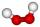How much distilled water (in liters) must pharmacists pour into 300 ml of 23.6% solution of hydrogen peroxide to get 2.7% solution to gargle?
• PeroxideHow many ml should we pour 30% of peroxide (H2O2) into 100ml H2O to give a 20% solution?
• SolutionsHow much 60% solution and how much 35% solution is needed to create 100 l of 40% solution?
• Sea waterSeawater contains about 4.3% salt. How many dm3 of distilled water we must pour into 5 dm3 of sea water to get water with 1.8% salt?
• Solutions, mixturesWe have 2 liters of 20% solution available. How much 70% solution do we need to add to it to get a 30% solution?
• Fertilizer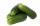Mr. Gherkin use for fertilization 7% solution of fertilizer. 17 liters of it still left. How much water must be added to the solution to make only 3% solution?
• SolutionWhat amount of 80% solution and what amount of water is needed to mix in order to obtain two liters of 60% solution of this mixture?
• Acid solutionBy adding 250 grams of a 96% sulfuric acid solution to its 3% solution, we changed its initial concentration to 25%. How many grams of 3% of the acid was used for dilution?
• Distilled waterSea water contains 5% salt. How many distilled water should be pour to 40 kg of sea water so that the salt content is 2%. How many kilograms of 2% of sea water we get?
• Mixture 2How many liters of water must be added to 7 liters of a 20% solution to obtain a 10% solution?
• Solutions, mixturesHow many liters of 70% solution we must add to 5 liters of 30% solution to give us a 60% solution?
• GramsHow many grams of 5% solution do we need to add to 100g of 50% solution to get a 20% solution?
• Water solutionHow many grams of salt should we dissolve in 400 g of water to get a 20% solution?
• SaltSeawater containing 5% salt. How many kg of freshwater necessary to pour into 40 kg of seawater so that the salinity fell to 2%?
• Copper sulphate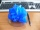How much g of water do we have to add to 240 g of an 84% CuSO4 solution to produce a 60% solution? (Express the mass of crystalline CuSO4 in the original solution and in the resulting solution and compare them. )
• Alcohol mixtureThree liters of 96 percent alcohol set up a certain amount of distilled water to form 54-percent alcohol. How many liters of distilled water was used?